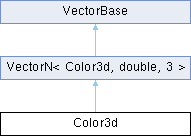MaterialX 1.38.7
Searching...
No Matches
Color3d Class Reference

A three-component color value (double-precision) More...

`#include <Types.h>`

Inheritance diagram for Color3d:## Public Member Functions

Color3d (double r, double g, double b)Public Member Functions inherited from VectorN< Color3d, double, 3 >
VectorN (Uninit)

VectorN (double s)

VectorN (const std::array< double, N > &arr)

VectorN (const vector< double > &vec)

VectorN (const double *begin, const double *end)

bool operator== (const Color3d &rhs) const
Return true if the given vector is identical to this one.

bool operator!= (const Color3d &rhs) const
Return true if the given vector differs from this one.

bool operator< (const Color3d &rhs) const
Compare two vectors lexicographically.

double & operator[] (size_t i)
Return the scalar value at the given index.

const double & operator[] (size_t i) const
Return the const scalar value at the given index.

Color3d operator+ (const Color3d &rhs) const

VectorNoperator+= (const Color3d &rhs)

Color3d operator- (const Color3d &rhs) const
Component-wise subtraction of two vectors.

Color3d operator- () const
Unary negation of a vector.

VectorNoperator-= (const Color3d &rhs)
Component-wise subtraction of two vectors.

Color3d operator* (const Color3d &rhs) const
Component-wise multiplication of two vectors.

Color3d operator* (double s) const
Component-wise multiplication of a vector by a scalar.

VectorNoperator*= (const Color3d &rhs)
Component-wise multiplication of two vectors.

VectorNoperator*= (double s)
Component-wise multiplication of a vector by a scalar.

Color3d operator/ (const Color3d &rhs) const
Component-wise division of two vectors.

Color3d operator/ (double s) const
Component-wise division of a vector by a scalar.

VectorNoperator/= (const Color3d &rhs)
Component-wise division of two vectors.

VectorNoperator/= (double s)
Component-wise division of a vector by a scalar.

double getMagnitude () const
Return the magnitude of the vector.

Color3d getNormalized () const
Return a normalized vector.

double dot (const Color3d &rhs) const
Return the dot product of two vectors.

Iterator begin ()

ConstIterator begin () const

Iterator end ()

ConstIterator end () const

double * data ()
Return a pointer to the underlying data array.

const double * data () const
Return a const pointer to the underlying data array.Public Types inherited from VectorN< Color3d, double, 3 >
using Iterator = typename std::array< double, N >::iterator

using ConstIterator = typename std::array< double, N >::const_iteratorStatic Public Member Functions inherited from VectorN< Color3d, double, 3 >
static constexpr size_t numElements ()
Return the number of scalar elements for the vector.Protected Attributes inherited from VectorN< Color3d, double, 3 >
std::array< double, N > _arr

## Detailed Description

A three-component color value (double-precision)

The documentation for this class was generated from the following file: